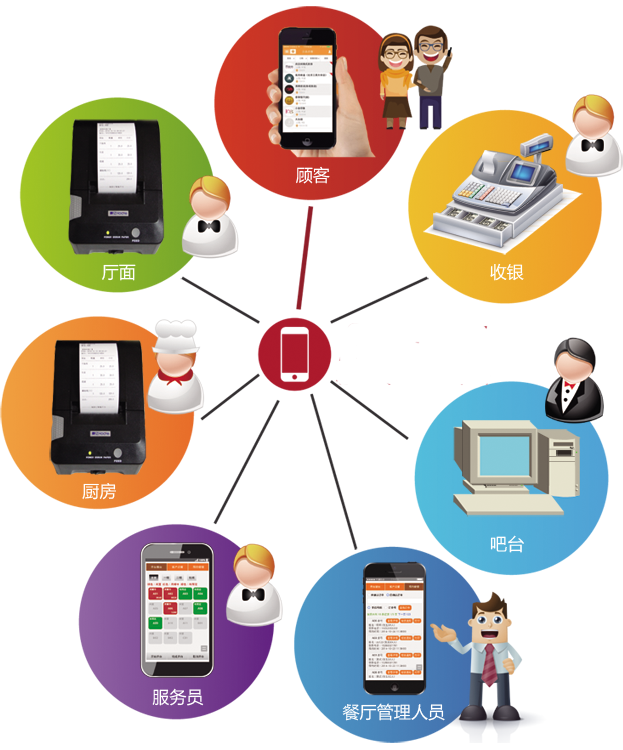客服电话：15825274336• 收银电脑点菜
• 手机微信点菜
• 立式点菜机
• 加菜
• 换菜
• 退菜
• 套餐点菜
• 餐标点菜

• 会员添加
• 会员分组
• 分组优惠设置
• 微信会员卡
• 会员储值
• 会员注销
• 会员统计
• 消费查询
• 微信会员充值
• ...

• 微信扫码支付
• 现金付款
• 刷卡付款
• 代金券
• 会员余额支付
• 折扣优惠
• 团购
• ...

• 物料材料管理
• 采购入库
• 销售出库
• 流水查询
• 库存盘点
• 成本统计
• ...

• 营业额走势图
• 客流量走势图
• 出品结构分析图
• 菜品销售统计
• 日结账单统计
• 营业日结账报表
• 营业月结账报表
• ...

• 员工添加
• 员工删除
• 权限设置
• 帐号设置
• 岗位管理
• 收银折扣权限配置
• ...

• 添加打印机
• 厨房分类出单设置
• 厨房总单分单设置
• 打印机启用停用
• 打印机类型设置
• ...

• 开台
• 撤台
• 转台
• 餐区管理
• 桌位管理
• 顺序调整

• 菜品添加
• 菜品编辑
• 菜品估清
• 分类管理
• 打印设置
• 顺序调整
• 折扣设置
• 图片介绍管理
• ...

• 数据备份
• 云升级
• 系统运行运监控

• 最新功能
• 吸粉营销
• O2O/餐饮
• 会员卡券
• 电商类
• 行业展示
• 教育培训
• 视频直播
• 社区

X